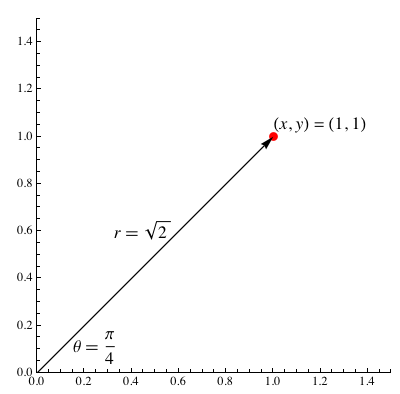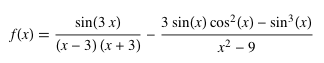AUTHOR PAGESam Blake
Blog Posts from this author:
August 3, 2011–

In my last blog post on plotting functionality in Wolfram|Alpha, we looked at 2D and 3D Cartesian plotting. In this post, we will look at 2D polar and parametric plotting.

For those of you unfamiliar with polar plots, a point on a plane in polar coordinates is located by determining an angle θ and a radius r. For example, the Cartesian point (x, y) = (1, 1) has the polar coordinates (r, θ) = (√2,π/4). The following diagram illustrates the relationship between Cartesian and polar plots.To generate a polar plot, we need to specify a function that, given an angle θ, returns a radius r that is a function r(θ). Making a polar plot in Wolfram|Alpha is very easy; for example, we can plot Archimedes’ spiral. More »

May 17, 2011–

Plotting functions in the Cartesian plane is such a simple task with Wolfram|Alpha: just enter the function you are looking to graph, and within seconds you will have a beautiful result. If you are feeling daring, enter a multivariate function, and the result will be a 3D Cartesian graph. Wolfram|Alpha is certainly not limited to Cartesian plotting; we have the functionality to make number lines, 2D and 3D polar plots, 2D and 3D parametric plots, 2D and 3D contour plots, implicit plots, log plots, log-linear plots, matrix plots, surface of revolution plots, region plots, list plots, pie charts, histograms, and more. Furthermore, in Wolfram|Alpha we can generate specialized plots for illustrating asymptotes, cusps, maxima, minima, inflection points, saddle points, solutions of ordinary differential equations, poles, eigenvalues, series expansions, definite integrals, 2D inequalities, interpolating polynomials, least-squares best fits, and more. Let’s take a look at the plotting functionality in Wolfram|Alpha, some of which is newly improved!

We will start simple with 2D Cartesian plots.

Here we plot sin(√7x)+19cos(x) for x between -20 and 20.

April 25, 2011–

Wolfram|Alpha is written in Mathematica, which as its name suggests is a fantastic system for doing mathematics. Strong algorithms for algebraic simplification have always been a central feature of computer algebra systems, so it should come as no surprise to know that Mathematica excels at simplifying algebraic expressions. The main two commands for simplifying an expression in Mathematica are Simplify and FullSimplify. There are also many specific commands for expressing an algebraic expression in some form. For example, if you want to expand a product of linear polynomials, Expand is the appropriate function.

The good news is that everyone has access to the power of Mathematica‘s simplification and algebraic manipulation commands in Wolfram|Alpha. We will now outline some of these features in Wolfram|Alpha, starting with the expression:and we will use Wolfram|Alpha to break it down to something significantly much simpler.
More »